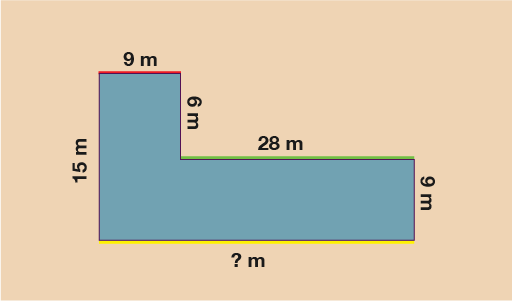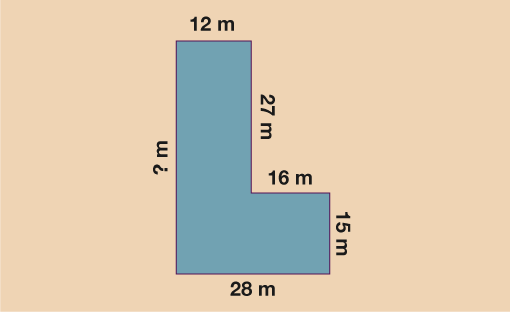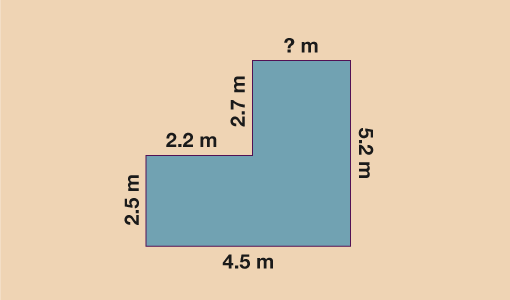Everyday maths 2 (Wales)

Start this free course now. Just create an account and sign in. Enrol and complete the course for a free statement of participation or digital badge if available.

Free course

# 1.2 Perimeters of shapes with missing lengthsFigure 7 Finding the perimeter when measurements are missing

Look at the shape above. You can see that one of the lengths is missing from the shape. How do you find the perimeter when you don’t have all the measurements? You cannot just assume that the missing length (yellow) is half of the red length, so how do you work it out? You’ll need to use the information that is given in the rest of the shape.

If you look at all the vertical lengths (red, yellow and green) you can see that we know the length of two of the three. You can also see that green + yellow = red, since the two shorter lengths put together would equal the same as the longest vertical length.

If 17 + ? = 30, then in order to find the missing length you must do 30 − 17 = 13.

The missing length is therefore 13 m. Now that you know this, you can work out the perimeter of the shape in the normal way.

• 30 + 12 + 13 + 20 + 17 + 32 = 124

• So the perimeter of this shape is 124 m.

Let’s look at one more example before you try some on your own.Figure 8 Finding the perimeter when a length is missing

In the example above, you will see that there is again a missing length. This time the missing length (yellow) is a horizontal one and so you need to look at the other two horizontal lengths (red and green) in order to work out the missing side.

You can see that this time that red + green = yellow, since the missing length is the sum of the two shorter lengths. You can now do 9 + 28 = 37, so the missing length is 37 m.

Now that you know all the side lengths, you can work out the perimeter by finding the total of all the lengths.

• 15 + 9 + 6 + 28 + 9 + 37 = 104

• So the perimeter of this shape is 104 m.

## Activity 2: Perimeters and missing lengths

1. Work out the perimeter of the shape below.Figure 9 Perimeters and missing lengths – Question 1
2. You are redecorating your living room and need to replace the skirting board. The layout of the room is shown below. Skirting board can only be bought in lengths of 2 m.

How many lengths should you buy?Figure 10 Perimeters and missing lengths – Question 2

1. Firstly, you need to work out the missing length:
• 15 + 27 = 42 m

So the perimeter is:

• 42 + 12 + 27 + 16 + 15 + 28 = 140 m
2. Again, work out the missing length first:
• 4.5 − 2.2 = 2.3 m

Next, work out the perimeter of the room:

• 2.5 + 2.2 + 2.7 + 2.3 + 5.2 + 4.5 = 19.4 m

To see how many 2 m length of skirting you will need do:

• 19.4 ÷ 2 = 9.7

Since we can only buy whole lengths we need to round this up to 10 lengths of skirting.

Good work! You can now work out perimeters of simple and complex shapes, including shapes where there are missing lengths. There is just one more shape you need to consider – circles.

Whether it’s ribbon around a cake or fencing around a pond, it’s useful to be able to work out how much of a material you will need to go around the edge of a circular shape. The final part of your work on perimeter focusses on finding the distance around the outside of a circle.

FSM_2_CYMRU#### Find out more

*Eligibility rules apply for financial support.